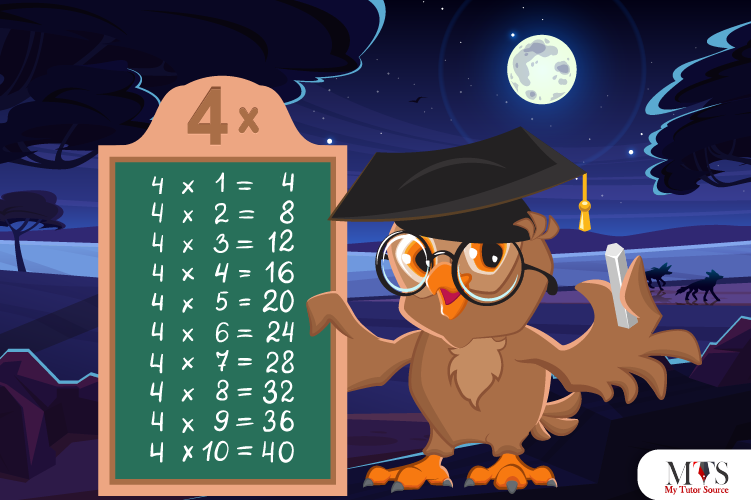# Multiplication Table of 4 – Tips to Memorize 4 Times Table & Example QuestionsTime to learn the multiplication table of 4. Another even number multiplication table like 2 times table. According to professors and private math tutors, memorizing even number tables is easy for some students. Remembering all multiplication tables not only helps you solve questions quickly or score higher but also helps you solve real-life queries in your mind. You could be at a shop buying 4 pairs of gloves. The cost of each team is \$10. That’s the time when knowledge of multiplication of 4 came to the rescue. You would simply multiply 4 with 10, and here is your bill. We do multiplication all day, every day, take a note.

In this article, we have covered everything related to the 4 times table. Let’s get started!

## Table of 4

Here is the complete table of 4 till 20 that needs to be memorized for calculating and solving problems within seconds. Don’t rush to learn the full table of 4 at once. Divide it into chunks like first learn it till 10 and then move forward.

4 x 1 = 4
4 x 2 = 8
4 x 3 = 12
4 x 4 = 16
4 x 5 = 20
4 x 6 = 24
4 x 7 = 28
4 x 8 = 32
4 x 9 = 36
4 x 10 = 40
4 x 11 = 44
4 x 12 = 48
4 x 13 = 52
4 x 14 = 56
4 x 15 = 60
4 x 16 = 64
4 x 17 = 68
4 x 18 = 72
4 x 19 = 76
4 x 20 = 80

## Method to Read Table of 4

In this section, we have given the multiplication table of 4 in words to let students know how to read it for learning purposes:

One time four is four (4)
Two times four is eight (8)
Three times four is twelve (12)
Four times four is sixteen (16)
Five times four is twenty (20)
Six times four is twenty-four (24)
Seven times four is twenty-eight (28)
Eight times four is thirty-two (32)
Nine times four is thirty-six (36)
Ten times four is forty (40)
Eleven times four is forty-four (44)
Twelve times four is forty-eight (48)
Thirteen times four is fifty-two (52)
Fourteen times four is fifty-six (56)
Fifteen times four is sixty (60)
Sixteen times four is sixty-four (64)
Four times four is sixty-eight (68)
Eighteen times four is seventy-two (72)
Nineteen times four is seventy-six (76)
Twenty times four is eighty (80)

## Tips for Memorizing Multiplication Table of 4

Everyone’s learning style is different, so the time required for learning also is. Some kids memorize tables in a few minutes only, whereas some take a couple of hours or days. It is normal. To fasten the process of learning and memorizing 4 times table, we have mentioned a few tips. Give them a try!

### Tip # 1: Even Pattern

Observe a little. The product of the 4 times table is always an even number, and its answers come with a pattern. Kids good with counting and even, odd, natural, or whole number differences can easily memorize the table of 4.

Here is a hint, if your answer of 4 times table multipliers is an odd number, it is undoubtedly wrong. Table of 4 has a pattern such as 4, 8, 12, 16, 20, 24, 28, and so on.

### Tip # 2: Learn with Objects

One of the exciting ways to memorize a table of 4 is using objects for learning. For instance, a chair has four legs and if you have 3 chairs, try counting the total number of legs. There are plenty of real-life examples that can be used to learn a table of 4. practicing such learning tips with students struggling with mathematics does wonders.

### Example Question of Table of 4

Question: How many legs do 8 dogs have?

As a dog has 4 legs
Therefore,
4 x 8 = 32
Thus, 8 dogs have 32 legs

Question: What does 4 x 5 is equal to?

4 x 5 is equal to 20

Question: Write a table of 4 from 6 to 9?

4 x 6 = 24
4 x 7 = 28
4 x 8 = 32
4 x 9 = 36

Question: What are 6 fours by addition and multiplication?

By addition: 4 + 4 + 4 + 4 + 4 + 4 = 24
By multiplication: 4 x 6 = 24

Question: What is the cost of 4 flowers if one flower is \$20?

Solution:

Cost of 1 flower = \$20
Cost of 4 flowers = 4 x 20 = 80
Hence, the cost of 4 flowers is \$80.

### Find Top Tutors in Your AreaWith over 3 years of experience in teaching, Chloe is very deeply connected with the topics that talk about the educational and general aspects of a student's life. Her writing has been very helpful for students to gain a better understanding of their academics and personal well-being. I’m also open to any suggestions that you might have! Please reach out to me at chloedaniel402 [at] gmail.com